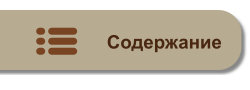MyTetra Share Делитесь знаниями!Functions
Время создания: 01.10.2017 14:54
Раздел: Go
Запись: xintrea/mytetra_db_mcold/master/base/1506858873k0g7msahls/text.html на raw.githubusercontent.com

A function is a group of statements that together perform a task. Every Go program has at least one function, which is main(). You can divide your code into separate functions. How you divide your code among different functions is up to you, but logically, the division should be such that each function performs a specific task.

A function declaration tells the compiler about a function name, return type, and parameters. A function definition provides the actual body of the function.

The Go standard library provides numerous built-in functions that your program can call. For example, the function len() takes arguments of various types and returns the length of the type. If a string is passed to it, the function returns the length of the string in bytes. If an array is passed to it, the function returns the length of the array.

Functions are also known as method, sub-routine, or procedure.

Defining a Function

The general form of a function definition in Go programming language is as follows −

`func function_name( [parameter list] ) [return_types]`
`{`
`   body of the function`
`}`

A function definition in Go programming language consists of a function header and a function body. Here are all the parts of a function −

• Func − It starts the declaration of a function.
• Function Name − It is the actual name of the function. The function name and the parameter list together constitute the function signature.
• Parameters − A parameter is like a placeholder. When a function is invoked, you pass a value to the parameter. This value is referred to as actual parameter or argument. The parameter list refers to the type, order, and number of the parameters of a function. Parameters are optional; that is, a function may contain no parameters.
• Return Type − A function may return a list of values. The return_types is the list of data types of the values the function returns. Some functions perform the desired operations without returning a value. In this case, the return_type is the not required.
• Function Body − It contains a collection of statements that define what the function does.

Example

The following source code shows a function called max(). This function takes two parameters num1 and num2 and returns the maximum between the two −

`/* function returning the max between two numbers */`
`func max(num1, num2 int) int {`
`   /* local variable declaration */`
`   result int`

`   if (num1 > num2) {`
`      result = num1`
`   } else {`
`      result = num2`
`   }`
`   return result `
`}`

Calling a Function

While creating a Go function, you give a definition of what the function has to do. To use a function, you will have to call that function to perform the defined task.

When a program calls a function, the program control is transferred to the called function. A called function performs a defined task and when its return statement is executed or when its function-ending closing brace is reached, it returns the program control back to the main program.

To call a function, you simply need to pass the required parameters along with its function name. If the function returns a value, then you can store the returned value. For example −

`package main`

`import "fmt"`

`func main() {`
`   /* local variable definition */`
`   var a int = 100`
`   var b int = 200`
`   var ret int`

`   /* calling a function to get max value */`
`   ret = max(a, b)`

`   fmt.Printf( "Max value is : %d\n", ret )`
`}`

`/* function returning the max between two numbers */`
`func max(num1, num2 int) int {`
`   /* local variable declaration */`
`   var result int`

`   if (num1 > num2) {`
`      result = num1`
`   } else {`
`      result = num2`
`   }`
`   return result `
`}`

We have kept the max() function along with the main() function and compiled the source code. While running the final executable, it would produce the following result −

`Max value is : 200`

Returning multiple values from Function

A Go function can return multiple values. For example −

`package main`

`import "fmt"`

`func swap(x, y string) (string, string) {`
`   return y, x`
`}`
`func main() {`
`   a, b := swap("Mahesh", "Kumar")`
`   fmt.Println(a, b)`
`}`

When the above code is compiled and executed, it produces the following result −

`Kumar Mahesh`

Function Arguments

If a function is to use arguments, it must declare variables that accept the values of the arguments. These variables are called the formal parametersof the function.

The formal parameters behave like other local variables inside the function and are created upon entry into the function and destroyed upon exit.

While calling a function, there are two ways that arguments can be passed to a function −

 Call Type Description Call by value This method copies the actual value of an argument into the formal parameter of the function. In this case, changes made to the parameter inside the function have no effect on the argument. Call by reference This method copies the address of an argument into the formal parameter. Inside the function, the address is used to access the actual argument used in the call. This means that changes made to the parameter affect the argument.

By default, Go uses call by value to pass arguments. In general, it means the code within a function cannot alter the arguments used to call the function. The above program, while calling the max() function, used the same method.

Function Usage

A function can be used in the following ways:

 Function Usage Description Function as Value Functions can be created on the fly and can be used as values. Function Closures Functions closures are anonymous functions and can be used in dynamic programming. Method Methods are special functions with a receiver.MyTetra Share v.0.58